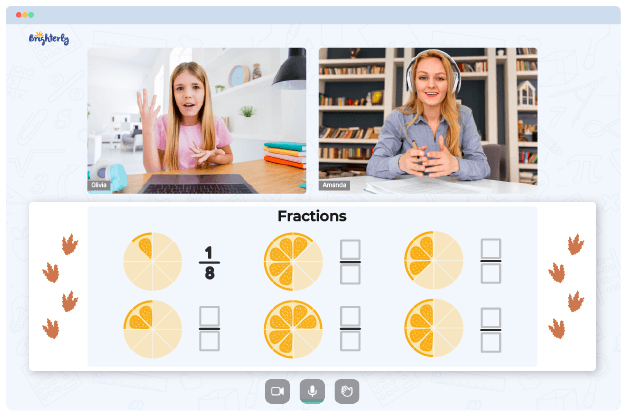# Reducing Fractions Worksheets

Reducing fractions problems are an excellent way to determine if a child can remember and recollect previously learned math concepts such as fractions and division. The more a child practices mixed fractions with worksheets, the more they improve math skills and become adept in handling advanced problems faster and accurately.

## Reducing Fractions to Lowest Terms Worksheets

A fraction can be simplified or reduced to the lowest terms when a numerator and denominator have a common factor.

There is an easy step a child can follow to reduce a fraction or simplify it. They should divide a numerator and denominator of a fraction by the most significant number possible for both numbers. In other words, the most significant standard number by which top and bottom numbers of a fraction can be divided should be identified.

There are two methods of solving problems from reducing fractions to lowest terms worksheets:

• Guess and check: Under this method, you pick a number that can divide evenly into both the fraction numerator and denominator, but in this case, you will not be sure if the number is exactly the largest. Therefore, you will continue to reduce the fraction further if necessary until you are left without any common factor.
• Greatest Common Factor (GCF): You will use the largest number to divide a fraction under this method.

Math for Kids

Is Your Child Struggling With Math?
1:1 Online Math TutoringYou arrive at the largest number by using factor trees to determine the prime factorization of a numerator and denominator.

And then, you will identify the GCF from your prime factorization. Please remember that after finding the Greatest Common Factor from a list of prime factors, you will pick the one with the fewest common elements.

Helping your kid with online math lessons for 4th grade. Expert tutors inspiring kids to love math so they can excel at It.

You can use both methods to practice exercises in a reducing fractions worksheet in PDF. Pick a technique that your child understands better to arrive at an answer. The guessing method will help a child simplify a fraction faster, while the GCF will help in yielding the lowest possible reduction.

### Benefits of Using Reducing Fractions Worksheets

Reducing fractions worksheets allow learners to complete numerous questions which are structured appropriately. Worksheets contain exercises of increasing difficulty levels, which assists kids in strengthening their knowledge of math concepts.

Reduce fractions worksheets also help students develop their problem-solving skills  because of the flexibility these tools offer. Therefore, even the youngest minds can practice and work at their own pace.### Reducing Fractions Worksheets PDF

Reducing Fractions Worksheet### Reducing Fractions Worksheets PDF

Reducing Fractions Worksheet PDF### Reducing Fractions Worksheets PDF

Reduce Fractions Worksheet### Reducing Fractions Worksheets PDF

Reduce Fractions Worksheets

These worksheets also allow students to practice reasoning and logical skills. Therefore, kids are prepared to handle real-life problems.

Due to visuals, learners can navigate through reducing fractions worksheets. A step-by-step approach allows kids to grasp concepts more effectively and solidify kids’ understanding of the topic.

### Fractions Worksheets

Need help with Fractions?• Does your child need extra assistance with understanding fractions?
• Start learning with an online tutor.

Is your child finding it hard to grasp fractions lessons? An online tutor could be of help.